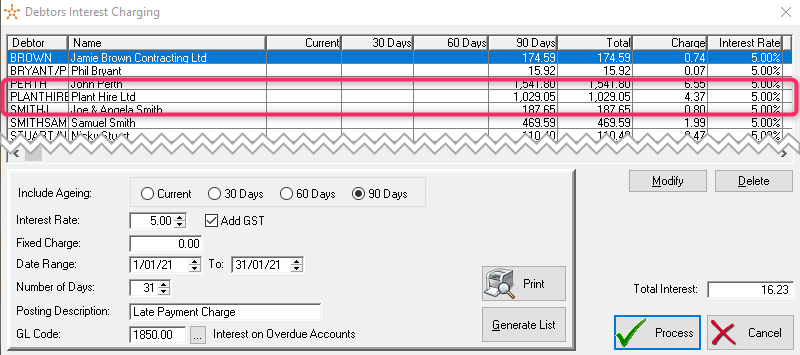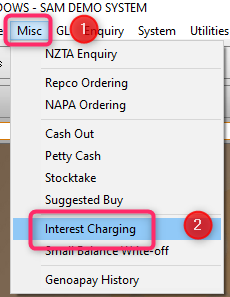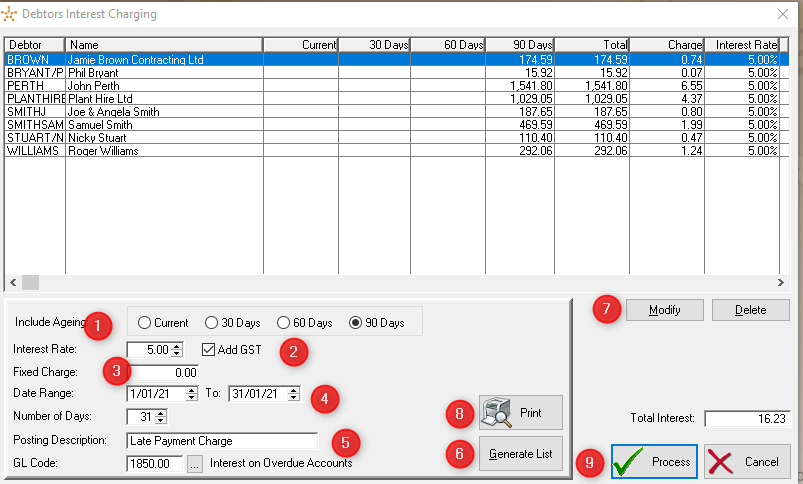How to Charge Interest and Fixed Charges to Customers Debtors Account using the Interest Charging Utility

# How Interest Is Calculated

The Interest Charge is calculated by applying the annual interest rate percentage to the specified balance, then dividing this value by 365 days and multiplying it by the number of days to be charged.  To this added any Fixed Charge and GST.

Overdue Amount / 365 Days x Days Interest Charged x Interest Rate = Interest Charged

## Example:So I have charged Plant Hire Ltd Interest on their outstanding balance of \$1029.05 so how was this 5% Interest Calculated?

1029.05 / 365 (days) = 2.82

2.82 x 31 (days interest charged) = 87.42

87.42 x 5% (Interest Rate Charged ) = 4.37

4.37 is amount of Interest to be charged to Customer

# Debtors Interest Charging1.  From the Main Sam Menu select Misc

2.  From the drop down menu select Interest Charging# Generate Debtors Interest Charging List

1. Select the Ageing of the Overdue Balances to be Included in the Interest Charging.

2. Select the Interest Rate and Add GST tick box.

3. The Fixed Charge is if an Overdue Fee is been charged at a Fixed Rate regardless of amount of Overdue Balance. This can also be used if Charging a monthly Account Fee.

4. Select the Date Range or Number of Days the Customer is been charged Interest.  This is normally the number of Days the has been Overdue and to be charged Interest for.

5.  This is the Posting Description and GL Code Code used for Interest.  Change these fields if required.

6. Select Generate List to display the List of Customers been Charged Interest.

7. Use the Modify and Delete options to Change the Customer List Displayed

8. Print the Debtors Interest Charging Report and confirm is correct.

8. Select Process which will add a Interest Charging Transaction to all Debtors Accounts for the Customers Displayed.

Didn’t find what you were looking for?

Our Support team are here to help, you can reach us by submitting a support ticket

or calling us on 09 583 2455